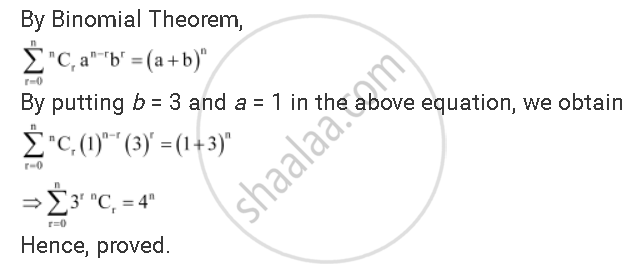CBSE (Arts) Class 11CBSE
Share
Notifications

View all notifications
Books Shortlist
Your shortlist is empty

# Binomial Theorem Prove that Sum_(R-0)^N 3^R ""^Nc_R = 4^N - CBSE (Arts) Class 11 - Mathematics

Login
Create free account

Forgot password?
ConceptBinomial Theorem for Positive Integral Indices

#### Question

Prove that sum_(r-0)^n 3^r  ""^nC_r = 4^n

#### SolutionIs there an error in this question or solution?

#### APPEARS IN

Solution Binomial Theorem Prove that Sum_(R-0)^N 3^R ""^Nc_R = 4^N Concept: Binomial Theorem for Positive Integral Indices.
S